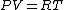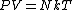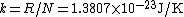# Boltzmann constant

(redirected from Boltzmanns constant)
Also found in: Dictionary, Medical.
Related to Boltzmanns constant: Joules, Wolfram Alpha

## Boltzmann constant

A constant occurring in practically all statistical formulas and having a numerical value of 1.3807 × 10-23 joule/K. It is represented by the letter k. If the temperature T is measured from absolute zero, the quantity kT has the dimensions of an energy and is usually called the thermal energy. At 300 K (room temperature) kT = 0.0259 electronvolt.

The value of the Boltzmann constant may be determined from the ideal gas law. For 1 mole of an ideal gas Eq. (1a)

(1a)(1b)holds, where P is the pressure, V the volume, and R the universal gas constant. The value of R, 8.31 J/K mole, may be obtained from equation-of-state data. Statistical mechanics yields for the gas law Eq. (1b). Here N, the number of molecules in 1 mole, is called Avogadro's number and is equal to 6.02 × 1023 molecules/mole. Hence, comparing Eqs. (1a) and (1b), one obtains Eq. (2). (2)Almost any relation derived on the basis of the partition function or the Bose-Einstein, Fermi-Dirac, or Boltzmann distribution contains the Boltzmann constant. See Boltzmann statistics, Bose-Einstein statistics, Fermi-Dirac statistics, Kinetic theory of matter, Statistical mechanics

McGraw-Hill Concise Encyclopedia of Physics. © 2002 by The McGraw-Hill Companies, Inc.

## Boltzmann constant

(bohlts -măn, -mahn) Symbol: k . A constant given by the ratio of the molar gas constant to the Avogadro constant and equal to 1.380 658 × 10–23 joules per kelvin. It is the constant by which the mean kinetic energy of a gas particle can be related to its thermodynamic temperature.
Collins Dictionary of Astronomy © Market House Books Ltd, 2006
The following article is from The Great Soviet Encyclopedia (1979). It might be outdated or ideologically biased.

## Boltzmann Constant

one of the fundamental physical constants; equal to the ratio of the universal gas constant R to Avogadro’s number NA (the number of molecules in 1 mole of a substance): k = R/NA. It is named for L. Boltzmann. The Boltzmann constant enters into a number of the major relations of physics—the equation of the state of an ideal gas and the expression for the average energy of the thermal motion of particles (and thermal capacity itself). It also connects the entropy of a physical system with its thermodynamic probability.

The Boltzmann constant is k = (1.380622 ± 0.000059) x 10-23 joules per °K; this value corresponds to the most accurate data (as of 1969) on the constants R and NA. The value of the Boltzmann constant can be determined directly, for example, by the experimental verification of the laws of radiation. [3–1572–1; updated]